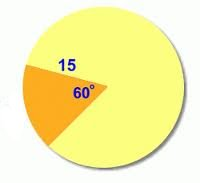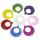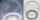# Circle and angle

What is the length of the arc of a circle with radius r = 207 mm with cental angle 5.33 rad?

d =  1103.3 mm
S =  114192.6 mm2

### Step-by-step explanation:Did you find an error or inaccuracy? Feel free to write us. Thank you!Tips to related online calculators
Do you want to convert length units?

#### You need to know the following knowledge to solve this word math problem:

We encourage you to watch this tutorial video on this math problem:

## Related math problems and questions:

• Arc and segmentCalculate the length of circular arc l, area of the circular arc S1 and area of circular segment S2. Radius of the circle is 11 and corresponding angle is (2)/(12) π.
• SectorThe perimeter of a circular sector with an angle 1.8 rad is 64 cm. Determine the radius of the circle from which the sector comes.
• Circle arcCircle segment has a circumference of 135.26 dm and 2096.58 dm2 area. Calculate the radius of the circle and size of the central angle.
• Arc-sectorarc length = 17 cm area of sector = 55 cm2 arc angle = ? the radius of the sector = ?
• Circle sectorThe circular sector with a central angle 160° has an area 452 cm2. Calculate its radius r.
• ArcWhat area of a circle occupied the flowers planted in the arc of a circle with radius 3 m with central angle 45°?
• Circle arcCalculate the circular arc area in m2 where the diameter is 263 dm, and a central angle is 40°. Result round to three decimal places.
• Length of the arcWhat is the arc length of a circle k (S, r=68mm), which belongs to a central angle of 78°?
• ArcCircle arc corresponding to angle is 32° is 28 dm long. What is the length of the entire circle?
• Circle sectionEquilateral triangle with side 33 is inscribed circle section whose center is in one of the vertices of the triangle and the arc touches the opposite side. Calculate: a) the length of the arc b) the ratio betewwn the circumference to the circle sector and
• Circular arcCalculate the length of the circular arc if the diameter d = 20cm and the angle alpha = 142 °
• ArcThe length of the circle is 41 amd arc length of the circle 9. What is the magnitude of the angle of this arc?
• Circular segmentCalculate the area S of the circular segment and the length of the circular arc l. The height of the circular segment is 2 cm and the angle α = 60°. Help formula: S = 1/2 r2. (Β-sinβ)
• Quarter circularThe wire that is hooked around the perimeter of the quarter-circular arc has length 3π+12. Determine the radius of the circle arc.Calculate the radius of the quadrant, which area is equal to area of circle with radius r = 15 cm.The irrigation sprinkler can twist with an angle of 320° and has a reach of 12 meters. Which area can irrigate?Calculate the circumference of a quarter circle if its content is S = 314 cm2.